# pi-DESIGNWhat is pi-DESIGN

pi-DESIGN is an application for advanced design of reinforced concrete elements (columns, beams, slabs) subjected to axial forces (N) and axial or biaxial (Mx and My) bending moments, according to EC2(EUROCODES).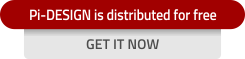What it does:

The application calculates the necessary areas of the reinforcing bars (As,req) , the R/C cross-sections strains, stresses, curvatures and stiffnesses and automatically generates the appropriate stress - deformation diagrams in both 2D and 3D environment.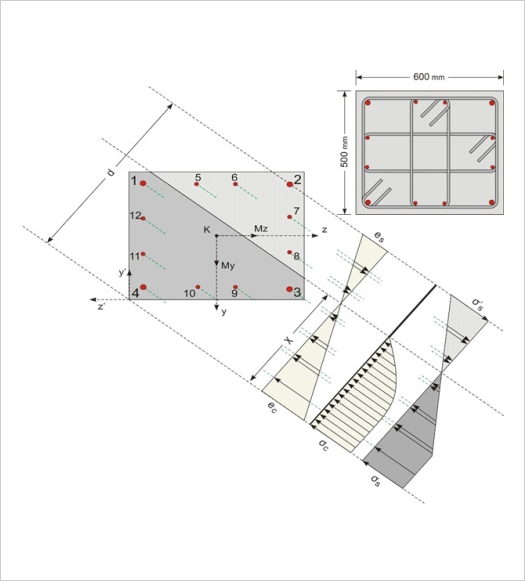The calculation of the neutral axis is performed with great precision.
The solution is also presented in 3D and Stereoscopic 3D (with the use of red-blue glasses).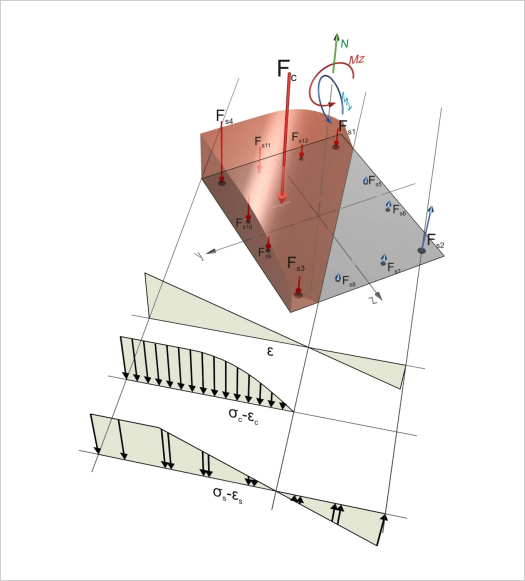The application supports:

- COLUMNS of sections: Rectangular, Gamma, Z-type, T-type, Circular, Quadrilateral, Π-type and elevator, with concentrated and distributed reinforcement, under uniaxial και biaxial bending.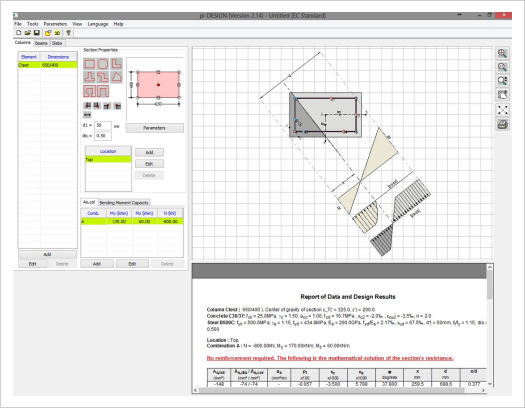C1, Combination A: Nd=-800kN, Myd=170kNm Mzd=60kNm As,cor=39.12cm2 [ρl=1.304%, εc=-3.50‰ εs=1.55‰, φ=52.07?, x=0.481m]

- BEAMS of sections: Rectangular, floor girder and I-type with tensile and compressive reinforcement bars.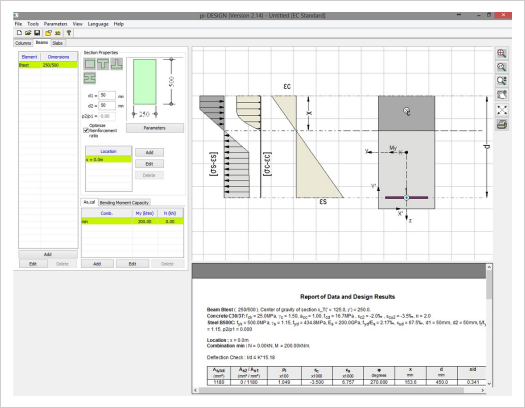B2, position: x=3.0m, Combination: max, Nd=-100kN Md=350kNm As,tot=25.77cm2 (As2/As1=3.26/22.51) [ρl=2.062%, εc=-3.50‰, εs=2.17‰, x/d=0.617]

- SLABS with single and double reinforcement.

•
•S1, Position: Span, Md=20.18kNm As=3.175cm2 [ρl=0.187%, εc=-3.50‰, εs=40.86‰, x/d=0.079]

- COMPATIBILITY:

• Bidirectional communication through standardized XML files.
• The application can import the reinforcement calculations for a specific floor or for the entire building.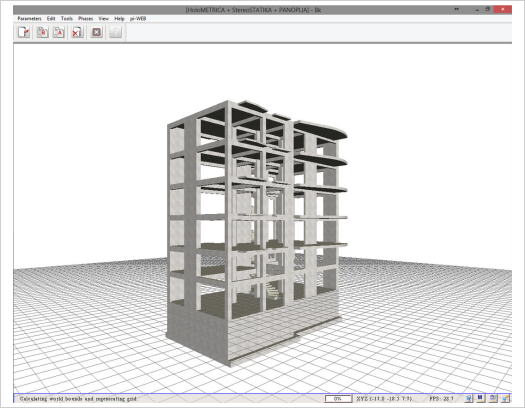Several applications (e.g. holoBIM) can create the necessary XML files for the communication with holoBIM

-REGULATIONS :EKOS 2000, EC2

- PARAMETRIC:

• Parametric generalized Integral Length Stress-Strain curve for concrete.
• Bilinear Stress-Strain Diagram for steel.

-Concrete grades :from C12 up to C90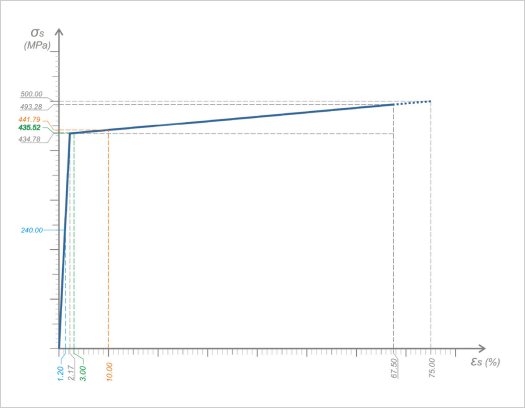Watch a video demonstration of pi-DESIGN application

The user guide analytically descibes the usage of the application and explains all the options available, with several photos and examples for easier comprehension. By clicking on the following link you can read online the user guide of pi-DESIGN.

The manual of the scientific documentation analytically explains the calculation methodology used in bending strength problems, in structural elements. The manual uses several practical examples in order to explain the usage of the application but mainly the structural bending problem.

BY clicking on the following link you can read online the scientific documentation of pi-DESIGN.